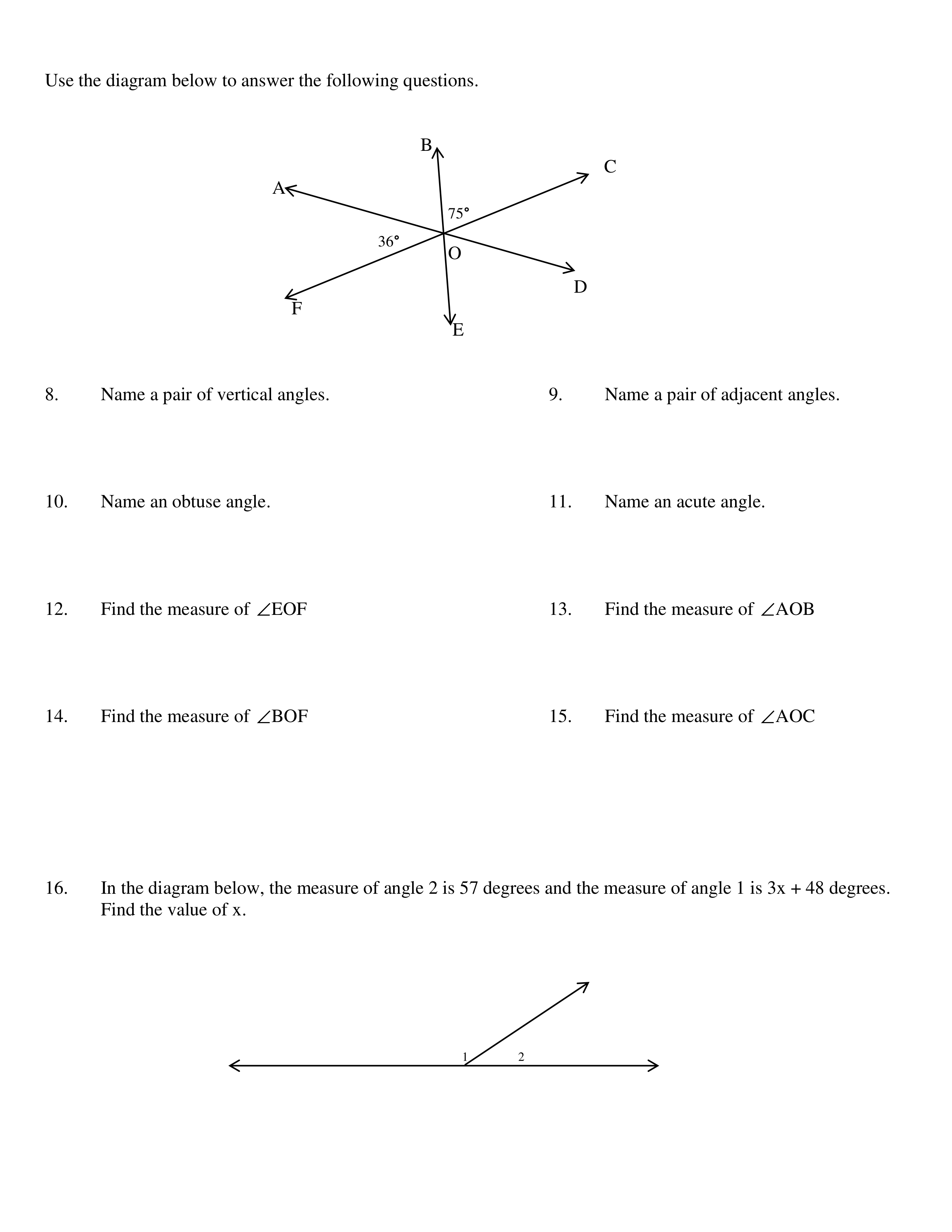Geometry Test Review
starstarstarstarstarstarstarstarstarstar
by Karen Kern
| 16 Questions1
2
3
4
5
6
7
1
1 pt
Find the value of x.
2
1 pt
Find the value of x.
3
1 pt
What is the measure of the third angle?
4
1 pt
Find the measure of angle BEC
5
1 pt
Determine the value of x.
6
1 pt
Find the value of x.
7
1 pt
Find the measurement of angle A8
9
10
11
12
13
14
15
16
8
1 pt
Name a pair of vertical angles
9
1 pt
Name a pair of adjacent angles
10
1 pt
Name an obtuse angle
11
1 pt
Name an acute angle
12
1 pt
Find the measure of angle EOF
13
1 pt
Find the measure of angle AOB
14
1 pt
Find the measure of angle BOF
15
1 pt
Find the measure of angle AOC
16
1 pt
Find the value of x.